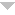orortakenwhy

Make sure to remember your password. If you forget it there is no way for StudyStack to send you a reset link. You would need to create a new account.

Enter the associated with your account, and we'll email you a link to reset your password.

# Zach Barna's waves, work, and power study stack.

Quiz yourself by thinking what should be in each of the black spaces below before clicking on it to display the answer.
Help

 QuestionAnswerWhat is a wave?   A disturbance that transfers energy in a medium.
What is a medium?   The material- solid, liquid, or gas- through which a wave can travel.
What is a transverse wave?   Particles of the medium move PERPENDICULAR compared to the direction the wave is moving.
What is the crest of a wave?   The highest point of the wave.
What is the trough of a wave?   The lowest point of the wave.
What is a longitudinal wave?   Particles of the medium move PARALLEL compared to the direction the wave is moving.
What is a compression?   When the waves come closer to one another.
What is a rarefaction?   When the waves spread farther apart from each other.
What are mechanical waves?   Waves that require a medium to pass through them.
What are electromagnetic waves?   Waves that don't require a medium to pass through them.
What is amplitude?   The measurement of how much energy is being carried by a wave. (This is determined by the distance between the crest and the resting position).
What is wavelength?   The distance from any point on the wave to the next identical point on the wave.
What is frequency?   The number of waves that pass a point in a given amount of time.
What are hertz (Hz)?   The measurement of frequency.
What is wave speed?   The time required for identical parts of waves to pass a given point. Speed= Wavelength x Frequency
What is force?   A push or a pull exerted on an object in order to change the motion of an object. Newtons are the unit of measurement.
What is work?   The use of force to move an object some distance. Joules are the unit of measurement. Work= Force x Distance
If a math book is 9 Newtons, and it is being pushed 3 meters, how much work is being done?   9 x 3 = 27 Joules
What is power?   The amount of work and force you use on an object. Watts are the unit of measurement. Power= Work/Time
If a bowling ball is 45 Newtons, and it is rolling down the end of a bowling lane, which is 18 meters and takes 5 seconds for the ball to reach the end, how much power is produced?   45 x 18 = 810 810/5 = 162 Watts
If the frequency of a wave was 100 Hz and the wavelength was 4 cm, how fast is it traveling?   100 x 4 = 400 cm/second
If a bag of chips is 2 Newtons, and it is thrown 45 meters across the backyard, how much work is being done?   2 x 45 = 90 Joules
If a weight is 20 Newtons, and it is pushed 15 meters across the floor, and is pushed for 6 seconds, how much power is produced?   20 x 15 = 300 300/6 = 50 Watts
If you are listening to music through your wireless speaker, where is the disturbance coming from?   The wireless speaker is the source of the disturbance.
Molly and Ed were doing a science lab together. They could not agree on an answer. "If the lion is roaring very loud, there is a high amplitude wave being produced," says Molly. Ed thinks differently. Is Molly correct, or should she listen to Ed? Why?   Molly is correct because a longitudinal wave does not have a crest or a trough, and if the lion is putting more energy into his roar, it will be louder, causing there to be more amplitude in the wave.
If you send a longitudinal wave ( yell ) at the wall in a very open room, what will happen?   The longitudinal wave you have sent at the wall will bounce it right back because it is not sending back any energy, causing it to bounce right off.
Jack had a theory when playing tug of war. If neither two people are moving/pulling the rope, he believed no work was being done. Is Jack correct or not? Why?   Yes, Jack is correct. Since work= force x distance, there has to be a force pulling on the rope, and since it is not moving, that does not apply. Also, if the rope is not being pulled any distance, there is no work being done.
John Jacob Jingle Heimer Schmidt believed that when he hits the baseball with a bat, he is not doing any work because it is just too easy for him. Is he correct or not? Why?   Noooooooo. Just because something is easy for him, as long as the ball is moving a distance, and there is a force applied to it ( which there is ) work is being done.

Embed Code - If you would like this activity on your web page, copy the script below and paste it into your web page.

Normal Size     Small Size show me how
Created by: 309238